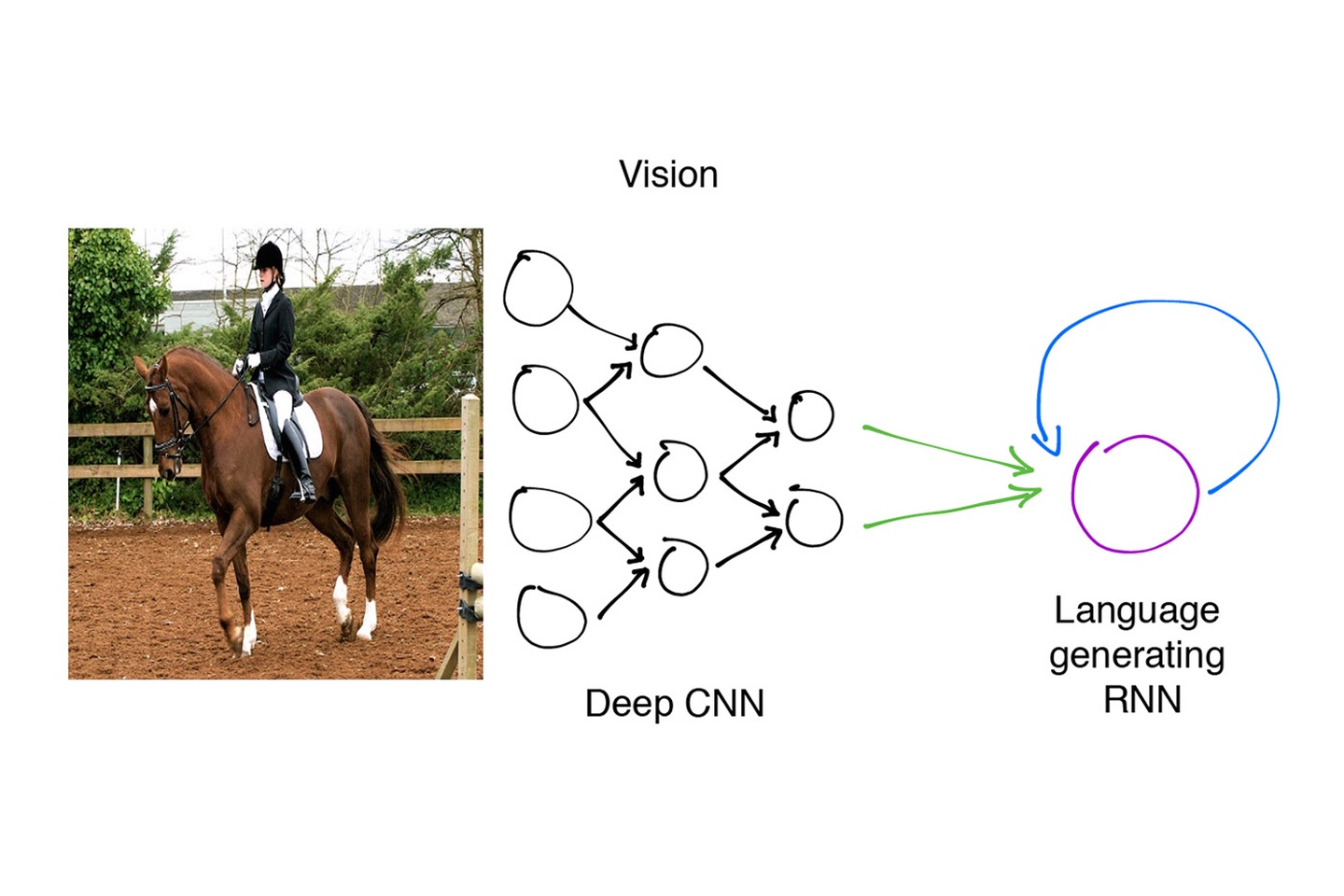`图1. 来源：Paul Puri。图片来自MS COCO数据集`

#### 图片字幕生成模型`图2. 来源：Shannon Shih获取自加州大学伯克利分校机器学习组织。马的图片来自MS COCO`

#### 字幕生成是图像分类的一种扩展

1. 在获取图片里的重要信息时，我们如何基于图像分类模型的成功结果？
2. 我们的模型如何能学习去融合对于语言的理解和对于图片的理解？

#### 利用迁移学习

def get_data(annotation_path, feature_path):

annotations = pd.read_table(annotation_path, sep=’\t’, header=None, names=[‘image’, ‘caption’])

return np.load(feature_path,’r’), annotations[‘caption’].values

#### 构建和训练这个模型`图3. 来源《Show and Tell：2015 MSCOCO图片字幕大赛所获得的经验教训》`

def build_model(self):

# declaring the placeholders for our extracted image feature vectors, our caption, and our mask

# (describes how long our caption is with an array of 0/1 values of length `maxlen`

img = tf.placeholder(tf.float32, [self.batch_size, self.dim_in])

caption_placeholder = tf.placeholder(tf.int32, [self.batch_size, self.n_lstm_steps])

mask = tf.placeholder(tf.float32, [self.batch_size, self.n_lstm_steps])

# getting an initial LSTM embedding from our image_imbedding

image_embedding = tf.matmul(img, self.img_embedding) + self.img_embedding_bias

# setting initial state of our LSTM

state = self.lstm.zero_state(self.batch_size, dtype=tf.float32)

total_ loss = 0.0

with tf.variable_scope(“RNN”):

for i in range(self.n_lstm_steps):

if i > 0:

#if this isn’t the first iteration of our LSTM we need to get the word_embedding corresponding

# to the (i-1)th word in our caption

with tf.device(“/cpu:0”):

current_embedding = tf.nn.embedding_lookup(self.word_embedding, caption_placeholder[:,i-1]) + self.embedding_bias

else:

#if this is the first iteration of our LSTM we utilize the embedded image as our input

current_embedding = image_embedding

if i > 0:

# allows us to reuse the LSTM tensor variable on each iteration

tf.get_variable_scope().reuse_variables()

out, state = self.lstm(current_embedding, state)

print (out,self.word_encoding,self.word_encoding_bias)

if i > 0:

#get the one-hot representation of the next word in our caption

labels = tf.expand_dims(caption_placeholder[:, i], 1)

ix_range=tf.range(0, self.batch_size, 1)

ixs = tf.expand_dims(ix_range, 1)

concat = tf.concat([ixs, labels],1)

onehot = tf.sparse_to_dense(

concat, tf.stack([self.batch_size, self.n_words]), 1.0, 0.0)

#perform a softmax classification to generate the next word in the caption

logit = tf.matmul(out, self.word_encoding) + self.word_encoding_bias

xentropy = tf.nn.softmax_cross_entropy_with_logits(logits=logit, labels=onehot)

xentropy = xentropy * mask[:,i]

loss = tf.reduce_sum(xentropy)

total_loss += loss

total_loss = total_loss / tf.reduce_sum(mask[:,1:])

return total_loss, img,  caption_placeholder, mask

#### 使用推断来生成字幕

def build_generator(self, maxlen, batchsize=1):

#same setup as `build_model` function

img = tf.placeholder(tf.float32, [self.batch_size, self.dim_in])

image_embedding = tf.matmul(img, self.img_embedding) + self.img_embedding_bias

state = self.lstm.zero_state(batchsize,dtype=tf.float32)

#declare list to hold the words of our generated captions

all_words = []

print (state,image_embedding,img)

with tf.variable_scope(“RNN”):

# in the first iteration we have no previous word, so we directly pass in the image embedding

# and set the `previous_word` to the embedding of the start token () for the future iterations

output, state = self.lstm(image_embedding, state)

previous_word = tf.nn.embedding_lookup(self.word_embedding, ) + self.embedding_bias

for i in range(maxlen):

tf.get_variable_scope().reuse_variables()

out, state = self.lstm(previous_word, state)

# get a one-hot word encoding from the output of the LSTM

logit = tf.matmul(out, self.word_encoding) + self.word_encoding_bias

best_word = tf.argmax(logit, 1)

with tf.device(“/cpu:0”):

# get the embedding of the best_word to use as input to the next iteration of our LSTM

previous_word = tf.nn.embedding_lookup(self.word_embedding, best_word)

previous_word += self.embedding_bias

all_words.append(best_word)

return img, all_words`图4 来源：Daniel Ricciardelli`

#### 局限和讨论`图5. 来源：Raul Puri，图片来自MS COCO数据集`

#### Raul Puri

Paul Puri是加州大学伯克利分校CO 2017届毕业的本科生研究人员。Raul已经对多个领域的研究项目做出了贡献，包括但不限于：机器人和自动化、计算机视觉、医疗成图、生物记忆设备等。不过所有这些研究工作都专注于机器学习和机器学习系统在安全、自主驾驶、自然语言处理、计算机视觉和机器人里面的应用。Raul也非常热情于通过教授应用机器学习概念课程来回馈社会。他还是多门伯克利分校机器学习课程的助教和讲师。

#### Daniel Ricciardelli

Dan Ricciardelli是加州大学伯克利分校的一名本科生研究员。他的研究方向包括用于金融和工业的自然语言处理、计算机视觉、深度主动学习和自动知识发现。Dan在伯克利机器学习组织里非常热心于让机器学习能为技术及非技术学生和专业人员所接触。The image caption generation model. (source: Shannon Shih from Machine Learning at Berkeley. Horse Image sourced from MS COCO.)